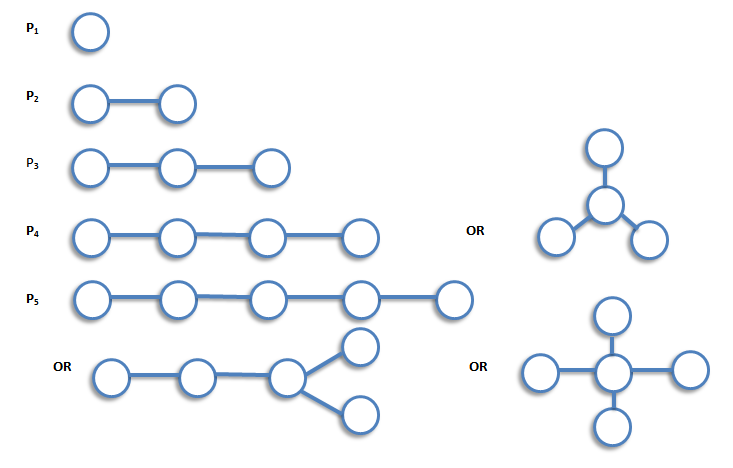Graph Theory

## Trees

An acyclic graph (also known as a forest) is a graph with no cycles. A tree is a connected acyclic graph. Thus each component of a forest is tree, and any tree is a connected forest.

Theorem    The following are equivalent in a graph G with n vertices.

1. G is a tree.
2. There is a unique path between every pair of vertices in G.
3. G is connected, and every edge in G is a bridge.
4. G is connected, and it has (n - 1) edges.
5. G is acyclic, and it has (n - 1) edges.
6. G is acyclic, and whenever any two arbitrary nonadjacent vertices in G are joined by and edge, the resulting enlarged graph G' has a unique cycle.
7. G is connected, and whenever any two arbitrary nonadjacent vertices in G are joined by an edge, the resulting enlarged graph has a unique cycle.

Generally speaking, algorithms associated with trees can be divided into three types.

• Algorithms for searching and labeling a given tree.
• Algorithms for constructing various types of tree.
• Algorithms for counting trees of a particular type.

Tree    A tree is a connected graph which contain no cycles.

For example,Theorem    Let T be a graph with n vertices. Then the following statements are equivalent.

1. T is connected and contains no cycles.
2. T is connected and has n-1 edges.
3. T has n-1 edges and contains no cycles.
4. T is connected and each edge is abridge.
5. Any two vertices of T are connected by exactly one path.
6. T contains no cycles, but the addition of any new edge creates exactly one cycles.